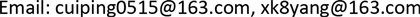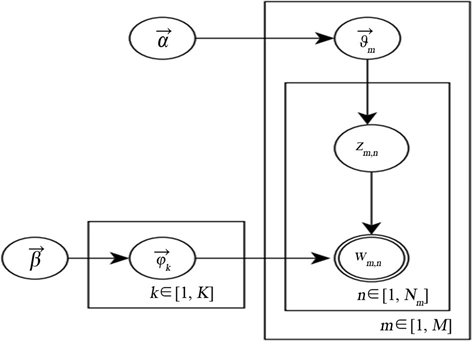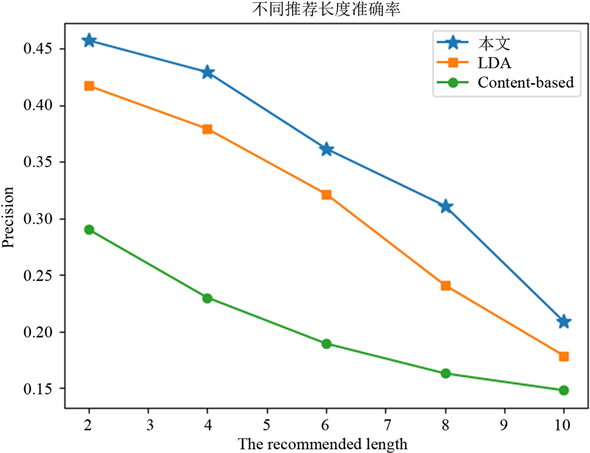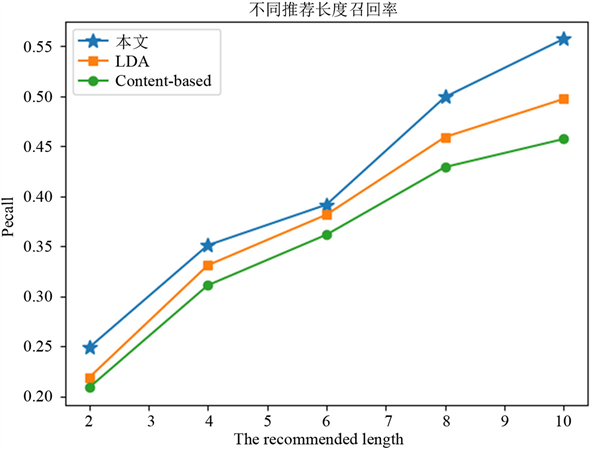﻿ 基于LDA主题扩展的个性化电影推荐系统 Personalized Movie Recommendation System Based on LDA Theme Extension

Computer Science and Application
Vol.08 No.06(2018), Article ID:25446,7 pages
10.12677/CSA.2018.86095

Personalized Movie Recommendation System Based on LDA Theme Extension

Ping Cui, Li Song, Xinkai Yang

College of Information, Mechanical and Electrical Engineering, Shanghai Normal University, ShanghaiReceived: May 24th, 2018; accepted: Jun. 8th, 2018; published: Jun. 15th, 2018ABSTRACT

Traditional movie recommendation algorithms based on user score data have some problems, such as sparse data and false score information, which cannot really and effectively express user interest. User comments, as an effective carrier of information from users’ interests and opinions, can quantify the product features through mining, analyzing and commenting information, and achieve personalized recommendation effect. Site review analysis of text information based on feature film recommended to the users in time according to the user history information analysis. User interest recommendation algorithm is proposed for expanding fusion sentiment analysis and LDA topic model features personalized selection keywords combined with TF-IDF weight item keywords and the characteristics development; the positive rate of sentiment analysis combined with topic comment expands the feature vector for item similarity calculation; the user is interested in the high similarity of the product as the recommended items list recommended. Experiments show that the proposed method improves the accuracy of recommendation.

Keywords:Recommendation System, Topic Model, Sentiment Analysis, Text Mining1. 引言

2. 相关研究

3. 基于情感分析和LDA主题拓展的推荐算法

3.1. 用户评论文本情感分析

Word2vec算法主要包含两种语言模型：CBOW (Continuous Bag of Words)和Skip-gram (Continuous Skip-gram Model)。这两种模型都包括输入层(input)、映射层(projection)、和输出层(output)。CBOW根据上下文语境预测目标词语，Skip-Gram根据当前单词预测上下文语境窗口内的词语。

1) 爬取评论信息经分词、去停用词等预处理；

2) 训练生成word2vec词向量模型；

3) 获取每条评论文本词语的词向量，计算每条评论文本词向量均值，做为每条评论文本的文本向量；

4) 将有标记的评论文本向量按4:1比例分为训练集和测试集，采用SVM分类器，筛选出物品的正向评论、获取物品正向评论率。物品i正向评论率 $pos{r}_{i}$ 为物品i正向评论条数 $coun{t}_{i}$ ，与物品i总评论条数 $comment{s}_{i}$ 比值。

3.2. LDA主题模型

LDA的图模型结构如上图1所示，在LDA模型中，一篇文档生成的方式如下：

1) 文档规模的大小服从Poisson分布，记作 $N\sim Possion\left(\xi \right)$

2) 文档 ${D}_{m}$ 主题分布参数的生成 ${\theta }_{m}\sim Dir\left(\alpha \right)$ ，即狄利克雷分布生成文档 ${D}_{m}$ 的K维主题向量 ${\theta }_{m}$ ，其中狄利克雷分布参数用 $\alpha$ 表示。这个操作需要重复N次，生成所有文档主题随机分布；

3) 生成文档特征词 ${w}_{m,n}$ ：以根据文档的主题向量 ${\theta }_{m}$ 的多项式分布 $Mulitinomial\left(\theta \right)$ 选择该词对应的某一隐藏主题 ${z}_{m,n}$ ，接着以多项式概率分布 $Mulitinomial\left(\phi \left(z\right)\right)$${\phi }_{k}$ ，从主题z中择某一特征词 ${w}_{m,n}$Figure 1. Three layer Bayesian network model diagram of LDA

3.3. LDA主题模型拓展

LDA主题模型对特征维度较高的评论词进行降维处理，对于评论文本信息，在使用LDA主题模型进行降维之后，可以获得每个文本关于主题的概率分布。记 ${D}_{i}\left({t}_{1},{t}_{2},\cdots ,{t}_{k}\right)$ 为电影i评论文本信息主题的概率分布。

$A\otimes B=R$ (1)

${Q}_{{D}_{i}}\otimes {T}_{{D}_{i}}^{\text{T}}={R}_{{D}_{i}}$${R}_{{D}_{i}}$ 即为文本 ${D}_{i}$ 的细粒度拓展主题关键词特征向量。

3.4. 个性化产品推荐列表

${w}_{i,j}=\frac{{s}_{i,j}}{{\sum }_{k=1}^{k=n}{s}_{i,k}}$ (2)

$sim\left(x,y\right)=\frac{1}{1+\sqrt{\sum {\left({x}_{i}-{y}_{i}\right)}^{2}}}$ (3)

$si{m}_{ij}=\frac{1}{1+d\left({D}_{i},{D}_{j}\right)}=\frac{1}{1+\sqrt{{\sum }_{s=1}^{s=K}{\left({p}_{i}{}_{,s}-{p}_{j,}{}_{s}\right)}^{2}+{\sum }_{s=1}^{s=MK}{\left({r}_{i,s}-{r}_{j,s}\right)}^{2}}}$ (4)

$si{m}_{ij}={w}_{i,j}\frac{1}{1+\sqrt{{\sum }_{s=1}^{s=K}u{t}_{s}{\left({p}_{i}{}_{,s}-{p}_{j,}{}_{s}\right)}^{2}+{\sum }_{s=1}^{s=MK}{\left({r}_{i,s}-{r}_{j,s}\right)}^{2}}}\cdot pos{r}_{i}$ (5)

4. 实验及结论Figure 2. Recommended precision under different recommended lengthsFigure 3. Recommended recall under different recommended lengths

Personalized Movie Recommendation System Based on LDA Theme Extension[J]. 计算机科学与应用, 2018, 08(06): 860-866. https://doi.org/10.12677/CSA.2018.86095

1. 1. Fang, B., Qiang, Y., Kucukusta, D., et al. (2016) Analysis of the Perceived Value of Online Tourism Reviews: Influence of Readability and Reviewer Characteristics. Tourism Management, 52, 498-506. https://doi.org/10.1016/j.tourman.2015.07.018

2. 2. Lee, Y.J., Hosanagar, K. and Tan, Y. (2015) Do I Follow My Friends or the Crowd? Information Cascades in Online Movie Ratings. Management Science, 61, 2241-2258. https://doi.org/10.1287/mnsc.2014.2082

3. 3. 安悦, 李兵, 杨瑞泰, 胡沥丹. 基于内容的热门微话题个性化推荐研究[J]. 情报杂志, 2014(2): 155-160.

4. 4. 单京晶. 基于内容的个性化推荐系统研究[D]: [硕士学位论文]. 长春: 东北师范大学, 2015.

5. 5. 李锋刚, 梁钰, Gao, X., 等. 基于LDA-WSVM模型的文本分类研究[J]. 计算机应用研究, 2015, 32(1): 21-25.

6. 6. 胡勇军, 江嘉欣, 常会友. 基于LDA高频词扩展的中文短文本分类[J]. 现代图书情报技术, 2013(6): 42-48.

7. 7. Chen, M., Jin, X. and Shen, D. (2011) Short Text Classification Improved by Learning Multi-Granularity Topics. Proceedings of the 22nd International Joint Conference on Artificial Intelligence, Barcelona, Catalonia, 16-22 July 2011, 1776-1781.

8. 8. Feng, S., Cao, J., Wang, J., et al. (2016) Group Recommendations Based on Comprehensive Latent Relationship Discovery. IEEE International Conference on Web Services, San Francisco, CA, 27 June-2 July 2016, 9-16.

9. 9. Aslanian, E., Radmanesh, M. and Jalili, M. (2016) Hybrid Recommender Sys-tems Based on Content Feature Relationship. IEEE Transactions on Industrial Informatics, 1-10.